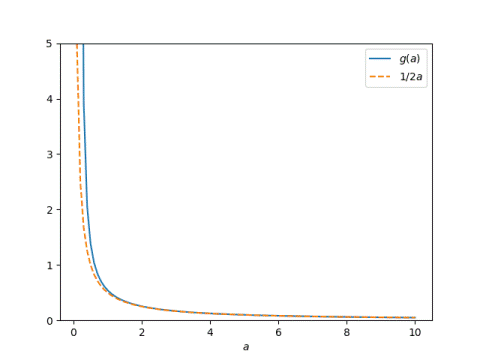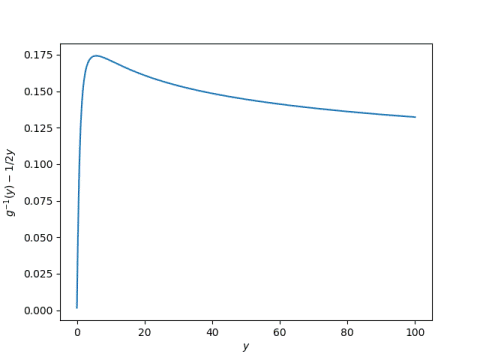# Solving for the catenary scale parameter

A catenary with scale a is the graph of the function

f(x; a) = a cosh(x/a) – a.

The x and the a are separated by a semicolon rather than a comma to imply that we think of x as the variable and a as a parameter.

This graph passes through the origin, i.e. for any a, f(0, a) = 0. To find the scaling parameter a we need to specify the value of f at one more point and solve f(x, a) = y. This is the equation I alluded to recently as not having a closed-form solution.

Without loss of generality, we can assume x = 1. Why is that?

Define

g(a) = f(1; a).

Then

f(x‘; a‘) = y

if and only if

g(a‘/x‘) = y‘/x‘.

So will assume x = 1 for now and focus on solving for a value of a such that g(a) = y. But we will include Python code shortly that goes back to f, i.e. does not assume x = 1.

The Taylor series for g looks like

g(a) = 1 / 2a + 1 / 24a³ + …

and so for large a,

g(a) ≈ 1 / 2a.

Here are a couple plots showing how good this approximation is, even for a not that large. First, a plot of g and its approximation.And here’s a plot of the relative error in approximating g(a) by 1/2a.This means that for small y,

g(1 / 2y) ≈ y.

It’s fortunate that we have a convenient approximation when y is small, because in practice y is usually small: catenaries are usually wider than deep, or at least not much deeper than wide.

Since the terms in the Taylor series that we discarded are all positive, we also have a bound

g(1 / 2y) > y.

If we want to solve for a numerically, 1 / 2y makes a good starting guess, and it also makes a left bracket for root-finding methods that require a bracket around the root.

Here’s Python code to solve f(x, a) = y for a, given x and y.

```    from numpy import cosh
from scipy.optimize import root

def g(a):
return a*cosh(1/a) - a

def solve_g(y):
assert(y > 0)
lower = 0.5/y
return root(lambda a: g(a) - y, lower).x

def solve_catenary(x, y):
"Solve for a such that a cosh(x/a) - a == y."
return x*solve_g(y/abs(x))
```

Now that we can solve g(a) = y numerically, we can go back and see how far 1 / 2y is from the actual solution for varying values of y.Recall that the second plot above showed that the relative error in approximating g(a) by 1 / 2a is large when a is small (and thus y is big). But the plot immediately above shows that nevertheless, 1/ 2y is a good guess at a solution to g(a) = y, never off by more than 0.175.

Recall also that we said 1 / 2y is a lower bound on the solution, and could be used as a left bracket in a root-finding method that requires a bracket. The plot above suggests that 0.18 + 1 / 2y would work as a right bracket